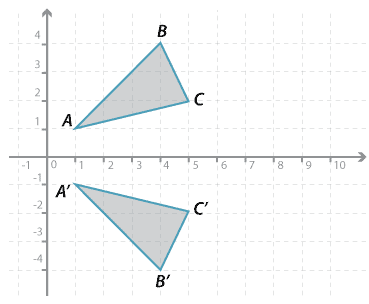#### Question 1Detailed description

Triangle $$ABC$$ is reflected to triangle $$A^\prime B^\prime C^\prime$$.

Give the $$x$$-coordinate and the $$y$$-coordinate of:

$$x$$ = $$y$$ = Submit answer
a $$A^\prime$$ ( , ).
b $$B^\prime$$ ( , ).
c $$C^\prime$$ ( , ).

Place answers in both checkboxes before submitting.

#### Question 2

The rectangle $$ABCD$$ is reflected in the $$x$$-axis to form rectangle $$A^\prime B^\prime C^\prime D^\prime$$. The coordinates of each of the vertices of $$ABCD$$ are as follows:

$$A(1,6); B(1,9); C(2,9); D(2,6)$$.

Give the $$x$$-coordinate and the $$y$$-coordinate of:

$$x$$ = $$y$$ = Submit answer
a $$A^\prime$$ ( , ).
b $$B^\prime$$ ( , ).
c $$C^\prime$$ ( , ).
d $$D^\prime$$ ( , ).

Place answers in both checkboxes before submitting.